# Can DBSCAN be a solution?¶

For Kmeans clustering to work well, the following assumptions have to hold true: :

• the variance of the distribution of each attribute (variable) is spherical

• all variables have the same variance

• the prior probability for all k clusters are the same, i.e. each cluster has roughly equal number of observations

If any one of these 3 assumptions is violated, then k-means does not do a good job.

Let's see with example data and explore if DBSCAN clustering can be a solution. I will show Kmeans with R, Python and Spark. Since Spark ML and Spark MLlib do not have DBSCAN algorithm, I will show DBSCAN with R and Python only.

PS: I have added hierarchical clustering with R at the end.

## Clusters with uneven sizes¶

As shown below, Kmeans does not work well with unevenly sized clusters.

In :
library(tidyverse)
library("fpc")
library(factoextra)
options(repr.plot.width=8, repr.plot.height=5)

set.seed(123)   #for reproducibility
sizes <- c(20, 100, 500)
centers <- data_frame(x = c(1, 4, 6), y = c(5, 0, 6), n = sizes,
cluster = factor(1:3))
df1 <- centers %>% group_by(cluster) %>%
do(data_frame(x = rnorm(.$n, .$x), y = rnorm(.$n, .$y)))

km<- kmeans(df1, centers= 3)
df1$cluster = as.character(km$cluster)
df1 %>% ggplot(aes(x = x, y = y, color  = cluster)) + geom_point() +
ggtitle('R: Kmeans of unevenly sized clusters') +
theme(axis.title = element_text(size=14), plot.title =
element_text(size = 16,colour="darkblue",hjust = 0.5))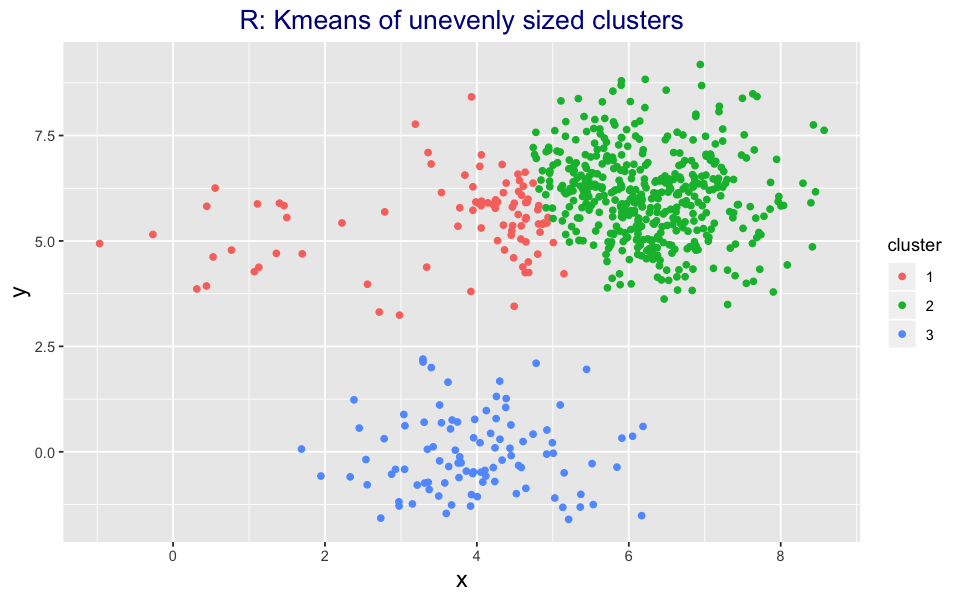In :
write.csv(df1 %>% select(x,y), 'df1.csv', row.names = FALSE)  # to try out the same data with Python and Spark

Adding missing grouping variables: cluster

In :
import pandas as pd
from sklearn.cluster import DBSCAN, KMeans
import matplotlib.pyplot as plt
%matplotlib inline

km = KMeans(n_clusters=3).fit(df1)
labels =  km.labels_
x = df1.values[:,0]
y = df1.values[:,1]
plt.figure(figsize=(14,7))
plt.scatter(x,y,c=labels,cmap='viridis',s=50,edgecolor='none')
plt.title('Python: Kmeans of unevenly sized clusters', fontsize = 20)
plt.show()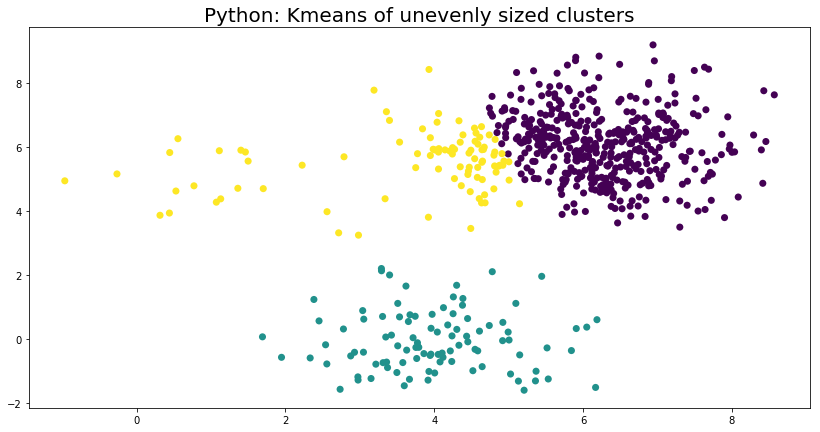In :
import findspark
findspark.init()
from pyspark.sql import SparkSession
from pyspark.ml.clustering import KMeans
from pyspark.sql import Row
spark = SparkSession.builder.getOrCreate()
from pyspark.ml.feature import VectorAssembler
import pyspark.sql.functions as f
from pyspark.ml.clustering import KMeans
from pyspark.sql.types import *

df1_spark = spark.createDataFrame(df1)
df1_spark = df1_spark.withColumn('Id', f.monotonically_increasing_id())
cols = df1_spark.drop('Id').columns
assembler = VectorAssembler(inputCols=cols, outputCol = 'features')
df1_spark_feature_vector = assembler.transform(df1_spark).select('Id', 'features')
kmeans = KMeans(featuresCol='features', predictionCol='prediction', k=3).setSeed(1)
model1 = kmeans.fit(df1_spark_feature_vector)
df1_clusters = model1.transform(df1_spark_feature_vector)

df1_spark_pandas = df1_spark.join(df1_clusters, df1_clusters.Id == df1_spark.Id, 'inner').select(['x','y','prediction']).toPandas()
cluster = df1_spark_pandas.values[:,2]
df1_spark_pandas.plot('x','y', kind = 'scatter', c = 'prediction', cmap='viridis', figsize=(14,8))
plt.title('PySpark: Kmeans of unevenly sized clusters', fontsize = 20)
plt.show()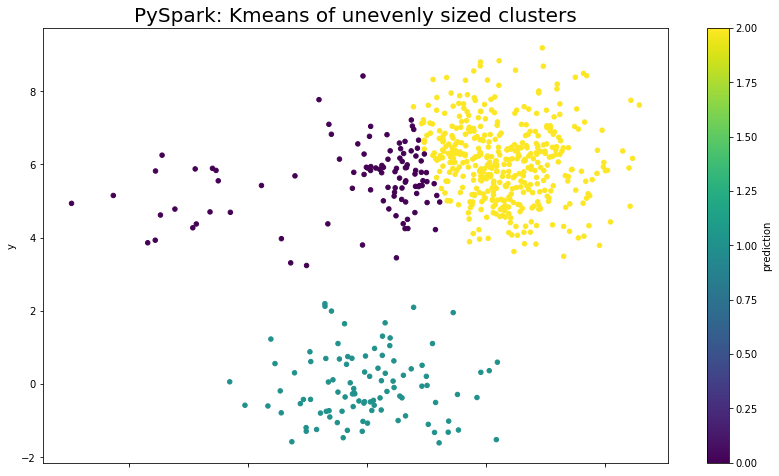## Non-spherical distribution¶

Since KMeans assumes spherical data distribution, as shown below, it does not work with non-spherical data.

In :
set.seed(134)
n <- 250
c1 <- data_frame(x = rnorm(n), y = rnorm(n))
c2 <- data_frame(r = rnorm(n, 5, .25), theta = runif(n, 0, 2 * pi),
x = r * cos(theta), y = r * sin(theta)) %>% dplyr::select(x, y)

df2 <- rbind(c1, c2)
km<- kmeans(df2, 2, nstart = 25)
df2$cluster = as.character(km$cluster)
df2 %>% ggplot(aes(x = x, y = y, color  = cluster)) + geom_point() +
ggtitle('R: Kmeans of non-spherical data') +
theme(axis.title = element_text(size=14), plot.title =
element_text(size = 16,colour="darkblue",hjust = 0.5))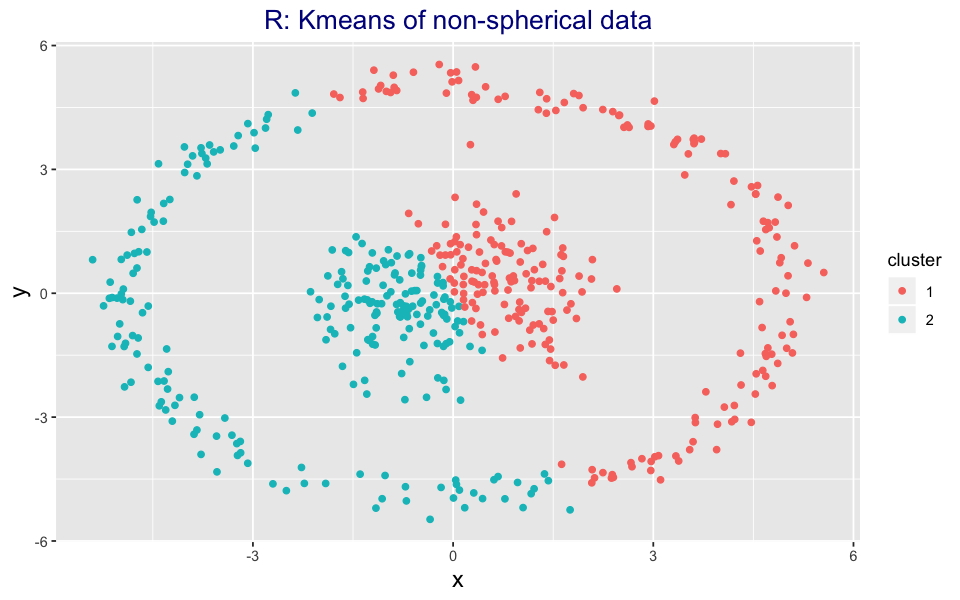In [ ]:
write.csv(df2 %>% select(x,y), 'df2.csv', row.names = FALSE)  # to try out the same data with Python and Spark

In :
df2 = pd.read_csv('df2.csv')
km = KMeans(n_clusters=2).fit(df2)
labels =  km.labels_
x = df2.values[:,0]
y = df2.values[:,1]
plt.figure(figsize=(14,7))
plt.scatter(x,y,c=labels,cmap='viridis',s=50,edgecolor='none')
plt.title('Python: Kmeans non-spherical data', fontsize = 20)
plt.show()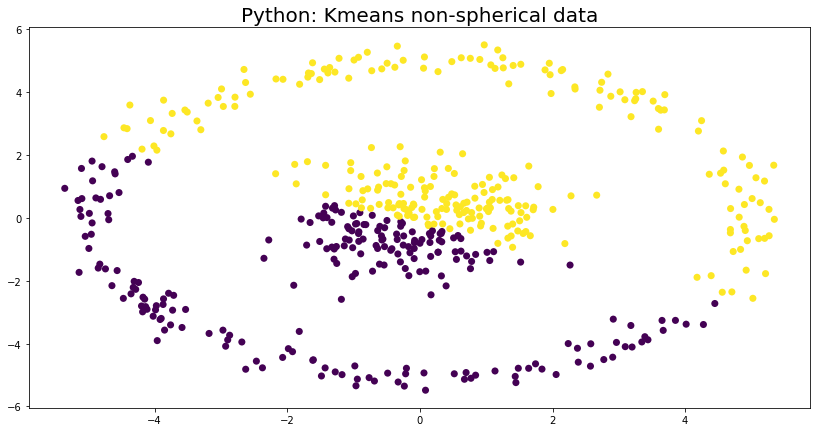In :
df2_spark = spark.createDataFrame(df2)
df2_spark = df2_spark.withColumn('Id', f.monotonically_increasing_id())
df2_spark_feature_vector = assembler.transform(df2_spark).select('Id', 'features')
kmeans = KMeans(featuresCol='features', predictionCol='prediction', k=2).setSeed(1)
model2 = kmeans.fit(df2_spark_feature_vector)
df2_clusters = model2.transform(df2_spark_feature_vector)

df2_spark_pandas = df2_spark.join(df2_clusters, df2_clusters.Id == df2_spark.Id, 'inner').select(['x','y','prediction']).toPandas()
df2_spark_pandas.plot('x','y', kind = 'scatter', c = 'prediction', cmap='viridis', figsize=(14,8))
plt.title('PySpark: Kmeans non-spherical data', fontsize = 20)
plt.show()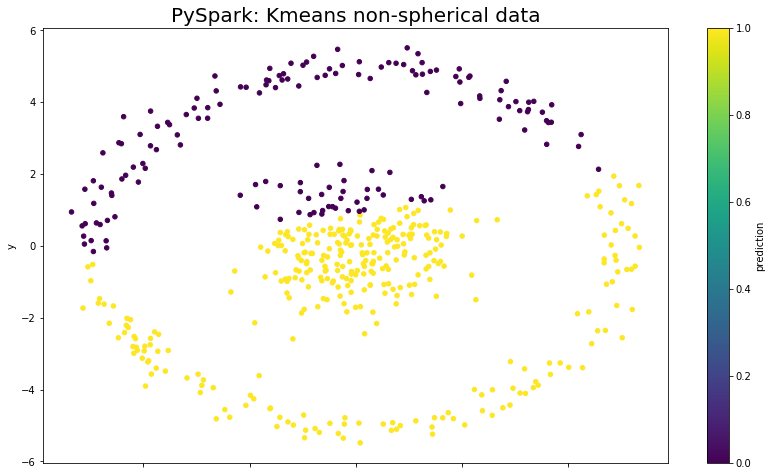## Non-spherical data with outliers¶

In :
data("multishapes")
df3 <- multishapes[, 1:2]
km<- kmeans(df3, 5, nstart = 25)
df3$cluster = as.character(km$cluster)
df3 %>% ggplot(aes(x = x, y = y, color  = cluster)) + geom_point() +
ggtitle('R: Kmeans of non-spherical data with noise') +
theme(axis.title = element_text(size=14), plot.title =
element_text(size = 16,colour="darkblue",hjust = 0.5))